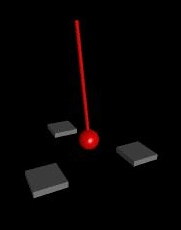QUESTION #437

# What is the chaos theory?For example, imagine an equilateral triangle made of wood with a strong magnet, positive pole facing up embedded at each vertex. suspend a magnet with negative pole facing down by a string exactly over the center of the triangle. If you move the pendulum to any point and then release it, the pendulum magnet will come to rest over one of the embedded magnets due to their mutual attraction. This simple physical system can be completely described by a few equations relating momentum, gravitational force, magnetic force, air resistance and such, and so you would think if you measured where the pendulum was before release you could accurately predict which of the 3 vertices it would end up with. But not so;